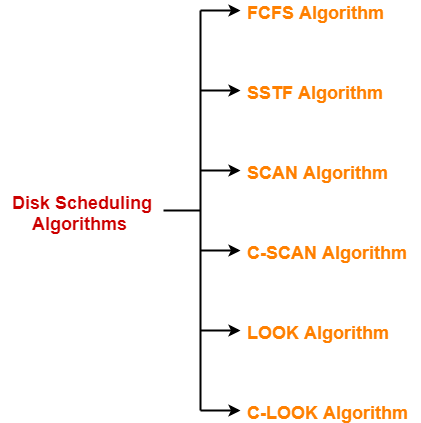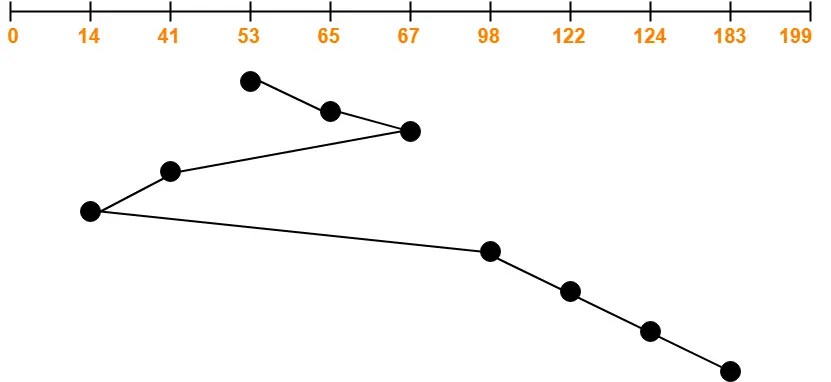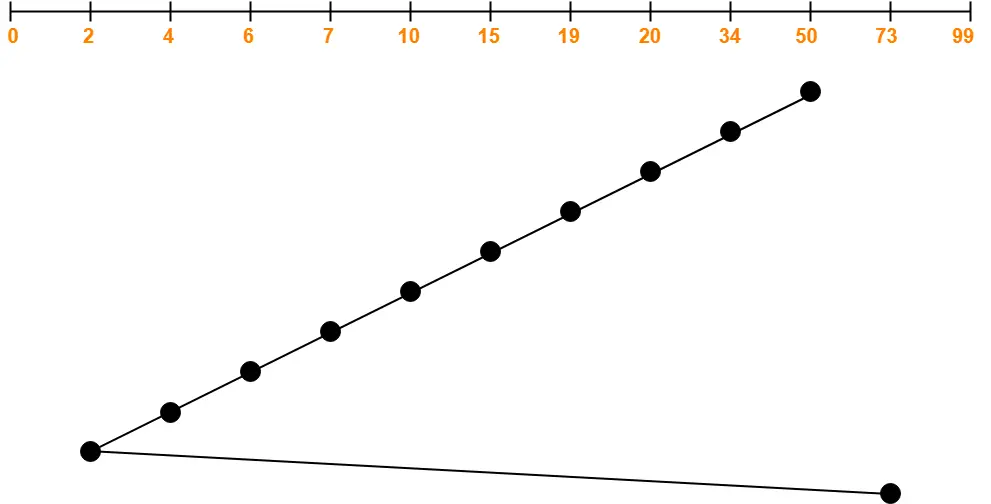# SSTF Algorithm | Disk Scheduling Algorithms

## Disk Scheduling Algorithms-

Before you go through this article, make sure that you have gone through the previous article on Disk Scheduling.

We have discussed-

• Disk scheduling algorithms are used to schedule multiple requests for accessing the disk.
• The purpose of disk scheduling algorithms is to reduce the total seek time.

Various disk scheduling algorithms are-## SSTF Disk Scheduling Algorithm-

• SSTF stands for Shortest Seek Time First.
• This algorithm services that request next which requires least number of head movements from its current position regardless of the direction.
• It breaks the tie in the direction of head movement.

• It reduces the total seek time as compared to FCFS.
• It provides increased throughput.
• It provides less average response time and waiting time.

• There is an overhead of finding out the closest request.
• The requests which are far from the head might starve for the CPU.
• It provides high variance in response time and waiting time.
• Switching the direction of head frequently slows down the algorithm.

## Problem-01:

Consider a disk queue with requests for I/O to blocks on cylinders 98, 183, 41, 122, 14, 124, 65, 67. The SSTF scheduling algorithm is used. The head is initially at cylinder number 53 moving towards larger cylinder numbers on its servicing pass. The cylinders are numbered from 0 to 199. The total head movement (in number of cylinders) incurred while servicing these requests is _______.

## Solution-Total head movements incurred while servicing these requests

= (65 – 53) + (67 – 65) + (67 – 41) + (41 – 14) + (98 – 14) + (122 – 98) + (124 – 122) + (183 – 124)

= 12 + 2 + 26 + 27 + 84 + 24 + 2 + 59

= 236

## Problem-02:

Consider a disk system with 100 cylinders. The requests to access the cylinders occur in following sequence-

4, 34, 10, 7, 19, 73, 2, 15, 6, 20

Assuming that the head is currently at cylinder 50, what is the time taken to satisfy all requests if it takes 1 ms to move from one cylinder to adjacent one and shortest seek time first policy is used?

1. 95 ms
2. 119 ms
3. 233 ms
4. 276 ms

## Solution-Total head movements incurred while servicing these requests

= (50 – 34) + (34 – 20) + (20 – 19) + (19 – 15) + (15 – 10) + (10 – 7) + (7 – 6) + (6 – 4) + (4 – 2) + (73 – 2)

= 16 + 14 + 1 + 4 + 5 + 3 + 1 + 2 + 2 + 71

= 119

Time taken for one head movement = 1 msec. So,

Time taken for 119 head movements

= 119 x 1 msec

= 119 msec

Thus, Option (B) is correct.

To gain better understanding about SSTF Disk Scheduling Algorithm,

Watch this Video Lecture

Next Article- SCAN Disk Scheduling Algorithm

Get more notes and other study material of Operating System.

Watch video lectures by visiting our YouTube channel LearnVidFun.

SummaryArticle Name
SSTF Algorithm | Disk Scheduling Algorithms
Description
SSTF Disk Scheduling Algorithm is a disk scheduling algorithm which services requests on the basis of shortest seek time first. SSTF Disk Scheduling Example. It reduces total seek time as compared to FCFS.
Author
Publisher Name
Gate Vidyalay
Publisher Logo